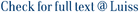We consider dynamic risk measures induced by backward stochastic differential equations (BSDEs) in an enlargement of filtration setting. On a fixed probability space, we are given a standard Brownian motion and a pair of random variables $( au, zeta) in (0,+infty) imes E$, with $E subset mathbb{R}^m$, that enlarge the reference filtration, i.e., the one generated by the Brownian motion. These random variables can be interpreted financially as a default time and an associated mark. After introducing a BSDE driven by the Brownian motion and the random measure associated to $( au, zeta)$, we define the dynamic risk measure $( ho_t)_{t in [0,T]}$, for a fixed time $T &gt; 0$, induced by its solution. We prove that $( ho_t)_{t in [0,T]}$ can be decomposed in a pair of risk measures, acting before and after $au$, and we characterize its properties giving suitable assumptions on the driver of the BSDE. Furthermore, we prove an inequality satisfied by the penalty term associated to the robust representation of $( ho_t)_{t in [0,T]}$ and we discuss the dynamic entropic risk measure case, providing examples where it is possible to write explicitly its decomposition and simulate it numerically.

Risk Measures and Progressive Enlargement of Filtration: A BSDE Approach / Calvia, Alessandro; Rosazza Gianin, Emanuela. - In: SIAM JOURNAL ON FINANCIAL MATHEMATICS. - ISSN 1945-497X. - 11:3(2020), pp. 815-848. [10.1137/19M1259134]

### Risk Measures and Progressive Enlargement of Filtration: A BSDE Approach

#### Abstract

We consider dynamic risk measures induced by backward stochastic differential equations (BSDEs) in an enlargement of filtration setting. On a fixed probability space, we are given a standard Brownian motion and a pair of random variables $( au, zeta) in (0,+infty) imes E$, with $E subset mathbb{R}^m$, that enlarge the reference filtration, i.e., the one generated by the Brownian motion. These random variables can be interpreted financially as a default time and an associated mark. After introducing a BSDE driven by the Brownian motion and the random measure associated to $( au, zeta)$, we define the dynamic risk measure $( ho_t)_{t in [0,T]}$, for a fixed time $T > 0$, induced by its solution. We prove that $( ho_t)_{t in [0,T]}$ can be decomposed in a pair of risk measures, acting before and after $au$, and we characterize its properties giving suitable assumptions on the driver of the BSDE. Furthermore, we prove an inequality satisfied by the penalty term associated to the robust representation of $( ho_t)_{t in [0,T]}$ and we discuss the dynamic entropic risk measure case, providing examples where it is possible to write explicitly its decomposition and simulate it numerically.
##### Scheda breve Scheda completa Scheda completa (DC)2020
risk measures, $g$-expectations, BSDEs, enlargement of filtration
Risk Measures and Progressive Enlargement of Filtration: A BSDE Approach / Calvia, Alessandro; Rosazza Gianin, Emanuela. - In: SIAM JOURNAL ON FINANCIAL MATHEMATICS. - ISSN 1945-497X. - 11:3(2020), pp. 815-848. [10.1137/19M1259134]
File in questo prodotto:
File
Calvia Rosazza Gianin - Risk meas and progr enl of filtr.pdf

Solo gestori archivio

Descrizione: Articolo
Tipologia: Versione dell'editore
Licenza: DRM (Digital rights management) non definiti
Dimensione 675.51 kB
Utilizza questo identificativo per citare o creare un link a questo documento: https://hdl.handle.net/11385/197075
•7
•5